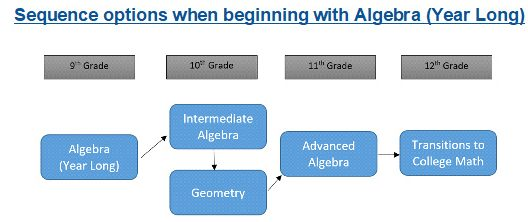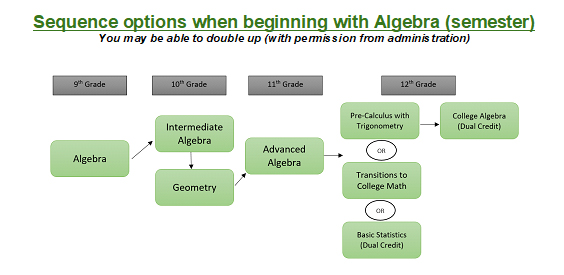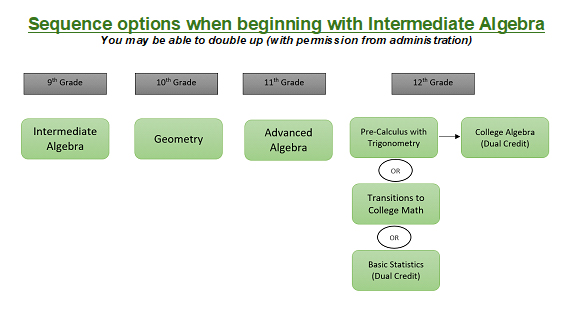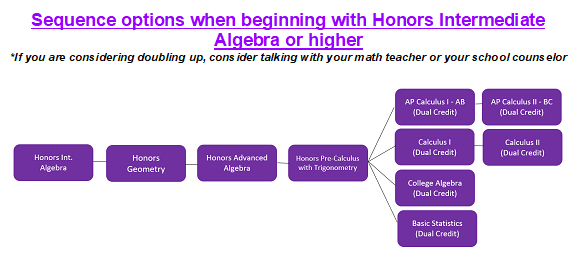# MATHEMATICS

Students are required to take 3 credits of mathematics to graduate. It is recommended that a student be enrolled in a mathematics course each year. Students will be placed into their initial courses upon entry into Batavia High School and freshmen are required to complete coursework through any level of Intermediate Algebra. Beginning sophomore year, students may elect to take two mathematics courses consecutively.Algebra (2 terms, 1 credit)

Prerequisite: Placement by current math teacher and counselor

Level: Fr

Fee: None – a graphing calculator (TI-84 or equivalent) is required.

Algebra is the foundation course of high school algebra with a focus on the study of variables and linear equations. Graphing techniques, abstract reasoning skills, and problem-solving skills are developed and applied to real-life situations. Algebra provides a strong base for future mathematics, science, and business-related courses. Topics include expressions, equations, inequalities, linear models, systems of equations and inequalities, exponents, polynomials, functions, factoring, sequences, and exponential representations.

Intermediate Algebra (2 terms, 1 credit)

Prerequisite: Algebra

Level: Fr

Fee: None – a graphing calculator (TI-84 or equivalent) is required.

Intermediate Algebra extends the study of algebra, its applications, and provides a bridge between Algebra and Advanced Algebra. The course makes regular use of the graphing calculator to make technology an integral part of the problem-solving process.

Topics include: functions, solving & graphing quadratics, advanced systems of equations, radicals expressions & equations, rational expressions & equations, probability, and statistics.

Intermediate Algebra Honors (2 terms, 1 credit)

Prerequisite: Algebra

Level: Fr

Fee: None – a graphing calculator (TI-84 or equivalent) is required.

Intermediate Algebra Honors extends the study of algebra, its applications, and provides a bridge between Algebra and Advanced Algebra Honors. The course makes regular use of the graphing calculator to make technology an integral part of the problem-solving process. The honors level course is for the student who shows exceptional mathematics ability and is willing to be challenged to think critically. Participation in the mathematics competition is highly encouraged.

Topics include: functions, solving & graphing quadratics, advanced systems of equations, radicals expressions & equations, rational expressions & equations, probability, and statistics.

Geometry (2 terms, 1 credit)

Prerequisite: Intermediate Algebra

Level: Fr, Soph

Fee: None – a graphing calculator (TI-84 or equivalent) is required.

Geometry deals with two- and three-dimensional figures and their properties. Basic terms to polygons and solids, visualization, pictorial representations, technology, and hands-on discovery are emphasized. Attention is given to developing logical thinking processes through various forms of proof. Algebra is strongly integrated into this course. Realistic and relevant applications of concepts and logical thinking processes are made to real-life problem settings.

Topics include: the tools of geometry, reasoning and proof, parallel and perpendicular lines, congruent triangles, relationships in triangles, quadrilaterals, proportions and similarity, trigonometry, circles, area, surface area, and volume.

Geometry Honors (2 terms, 1 credit)

Prerequisite: Intermediate Algebra Honors

Level: Fr, Soph

Fee: None – a graphing calculator (TI-84 or equivalent) is required.

Geometry Honors deals with two- and three-dimensional figures and their properties. Basic terms to polygons and solids, visualization, pictorial representations, technology, and hands-on discovery are emphasized. Attention is given to developing logical thinking processes through various forms of proof. Algebra is strongly integrated into this course in solving geometry problems. Realistic and relevant applications of concepts and logical thinking processes are made to real-life problem settings. The honors level course is for the student who shows exceptional mathematics ability and is willing to be challenged to think critically. Participation in the mathematics competition is highly encouraged.

Topics include: the tools of geometry, reasoning and proof, parallel and perpendicular lines, congruent triangles, relationships in triangles, quadrilaterals, proportions and similarity, trigonometry, circles, area, surface area, and volume.

Advanced Algebra (2 terms, 1 credit)

Prerequisite: Geometry

Level: Soph, Jr, Sr Elective

Fee: None – a graphing calculator (TI-84 or equivalent) is required.

Advanced Algebra is designed to prepare students for higher-level math courses. The course makes regular use of the graphing cal­culator to make technology an integral part of the problem-solving process. Topics include functions, complex numbers, polynomials, ra­tional expressions and equations, and exponential and logarithmic relations.

Advanced Algebra Honors (2 terms, 1 credit)

Prerequisite: Geometry Honors

Level: Soph, Jr Elective

Fee: None – a graphing calculator (TI-84 or equivalent) is required.

Advanced Algebra Honors is the capstone algebra course designed to prepare college-bound students for college entrance exams and other higher-level math courses. The course makes regular use of the graphing calculator to make technology an integral part of the problem-solving process. The honors level course is for the student who shows exceptional mathematics ability and is willing to be challenged to think critically. Participation in the mathematics competition is highly encouraged.

Topics include: complex numbers and radicals, quadratic structure, advanced systems of equations & inequalities, rational exponents, rational expressions & equations, functions, graphing & solving polynomials equations with degree greater than 2, properties of exponential functions & logarithmic functions, solving logarithmic & exponential functions, and conic sections.

Transitions to College Math (1 Sem, 1 Credit)

Prerequisite: 3 Math Credits

Level: Sr

Fee: None – a graphing calculator (TI-84 or equivalent) is required.

Transitions to College Mathematics is designed for those students who need additional preparation and review in order to be college and career-ready in mathematics.  The course is specifically designed to help prepare students for college placement exams that are used at most colleges and universities to determine what courses freshmen may register for.

Precalculus with Trigonometry (2 terms, 1 credit)

Level: Soph, Jr, Sr Elective

Fee: None – a graphing calculator (TI-84 or equivalent) is required.

Precalculus and Trigonometry is designed to prepare college-bound students for Calculus and other higher-level math courses. The course makes regular use of the graphing calculator to make technology an integral part of the problem-solving process.

Topics include: trigonometric functions, trigonometric equations, trigonometric applications, triangle trigonometry, trigonometric addition formulas, polar coordinates, complex numbers, vectors, sequences and series, and probability.

Precalculus with Trigonometry Honors (2 terms, 1 credit)

Level: Soph, Jr, Sr Elective

Fee: None – a graphing calculator (TI-84 or equivalent) is required.

Precalculus and Trigonometry Honors is designed to prepare college-bound students for Calculus and other higher-level math courses. The course makes regular use of the graphing calculator to make technology an integral part of the problem-solving process. The honors level course is for the student who shows exceptional mathematics ability and is willing to be challenged to think critically. Participation in the mathematics competition is highly encouraged.

Topics include: trigonometric functions, trigonometric equations, trigonometric applications, triangle trigonometry, trigonometric addition formulas, polar coordinates, complex numbers, vectors, limits, and derivatives.

Calculus I (2 terms, 1 credit, 4 credit hours at WCC)

Prerequisite: Precalculus with Trigonometry

Level: Jr, Sr Elective

Fee: A \$32 fee will be assessed should the student elect to pursue dual credit.  A graphing calculator (TI-84 or equivalent) is required.

Calculus I is equivalent to the content in the first course of a three-semester college calculus sequence. The course makes regular use of the graphing calculator to make technology an integral part of the problem-solving process.  Topics include limits, differentiation, applications of differentiation, integration, applications of integration, and transcendental functions.  Students enrolled in this course will have the option to receive Dual Credit for this course from Waubonsee Community College.  This course is the equivalent of MTH 131 at WCC and is an Illinois Articulated Course.  Students and parents pursuing Dual Credit should confer with their potential college choices about the transferability of this course.

Calculus II (2 terms, 1 credit, 4 credit hours at WCC)

Prerequisite: Calculus I

Level: Sr Elective

Fee: A \$32 fee will be assessed should the student elect to pursue dual credit.  A graphing calculator (TI-84 or equivalent) is required.

Calculus II is equivalent to the content in the second course of a three-semester college calculus sequence. The course makes regular use of the graphing calculator to make technology an integral part of the problem-solving process.   Topics include exponential and logarithmic functions, calculus of trigonometric functions, volumes and other applications of integration, formal integration techniques, indeterminate forms, L’Hopitals rule, improper integrals, sequences and series, convergence tests, Taylor’s formula, Taylor and Maclaurin series.  Students enrolled in this course will have the option to receive Dual Credit for this course from Waubonsee Community College.  This course is the equivalent of MTH 132 at WCC and is an Illinois Articulated Course.  Students and parents pursuing Dual Credit should confer with their potential college choices about the transferability of this course.

AP Calculus I (AB) (2 terms, 1 credit, 4 credit hours at WCC)

Prerequisite: Precalculus with Trigonometry Honors

Level: Jr, Sr Elective

Fee: \$35.50 – an additional \$32 fee will be assessed should the student elect to pursue dual credit.  A graphing calculator (TI-84 or equivalent) is required.

AP Calculus I (AB) is equivalent to the content in the first course of a three-semester college calculus sequence and prepares students to take the Advanced Placement – Calculus AB test. The course makes regular use of the graphing calculator to make technology an integral part of the problem-solving process. The AP level course is for the student who shows exceptional mathematics ability and is willing to be challenged to think critically. Topics include limits, differentiation, applications of differentiation, integration, applications of integration, and transcendental functions.  Students enrolled in this course will have the option to receive Dual Credit for this course from Waubonsee Community College.  This course is the equivalent of MTH 131 at WCC and is an Illinois Articulated Course.  Students and parents pursuing Dual Credit should confer with their potential college choices about the transferability of this course.

AP Calculus II (BC) (2 terms, 1 credit, 4 credit hours at WCC)

Prerequisite: AP Calculus I (AB)

Level: Sr Elective

Fee: \$37.50 – an additional \$32 fee will be assessed should the student elect to pursue dual credit.  A graphing calculator (TI-84 or equivalent) is required.

AP Calculus II (BC) is a second course in calculus and analytic geometry, and is a continuation of AP Calculus I (AB) and prepares students to take the Advanced Placement – Calculus BC test. The course makes regular use of the graphing calculator to make technology an integral part of the problem-solving process. The AP level course is for the student who shows exceptional mathematics ability and is willing to be challenged to think critically. Topics include exponential and logarithmic functions, calculus of trigonometric functions, volumes and other applications of integration, formal integration techniques, indeterminate forms, L’Hopitals rule, improper integrals, sequences and series, convergence tests, Taylor’s formula, Taylor and Maclaurin series. Students enrolled in this course will have the option to receive Dual Credit for this course from Waubonsee Community College.  This course is the equivalent of MTH 132 at WCC and is an Illinois Articulated Course.  Students and parents pursuing Dual Credit should confer with their potential college choices about the transferability of this course.

College Algebra: Dual Credit (2 terms, 1 credit at BHS, 3 credit hours at WCC)

Prerequisite: Successful completion of Precalculus with Trigonometry and a qualifying score on either SAT (530) or ACT (22)

Level: Sr Elective

Fee: A \$24 fee will be assessed should the student elect to pursue dual credit.  A graphing calculator (TI-84 or equivalent) is required.

This college-level course is designed to provide the students with algebraic concepts necessary to move into a stem-driven mathematics program. Topics include: real numbers, complex numbers, solutions of inequalities and equations, coordinate systems, functions, polynomials, rational functions, exponential and logarithmic functions, graphing and transformations of functions, and systems of equations.

Students enrolled in this course will have the option to receive Dual Credit for this course from Waubonsee Community College.  This course is the equivalent of MTH 129 at WCC.  Students and parents pursuing Dual Credit should confer with their potential college choices about the transferability of this course.

Basic Statistics: Dual Credit (2 terms, 1 credit at BHS, 3 credit hours at WCC)

Prerequisite: Successful completion of Advanced Algebra or higher and a qualifying score on either the SAT (530) or ACT (22)

Level: Jr, Sr Elective

Fee: A \$24 fee will be assessed should the student elect to pursue dual credit.  A graphing calculator (TI-84 or equivalent) is required.

This college-level course focuses on mathematical reasoning and the solving of real-life problems, rather than on routine skills and appreciation. This course in mathematics is designed to assist the student in the understanding and use of numerical data. Course content includes descriptive methods, probability, probability distributions, statistical inference, confidence intervals, tests of hypotheses, and correlation and regression. Students enrolled in this course will have the option to receive Dual Credit for this course from Waubonsee Community College. This course is the equivalent of MTH 107 at WCC and is an Illinois Articulated Course. Students and parents pursuing Dual Credit should confer with their potential college choices about the transferability of this course.